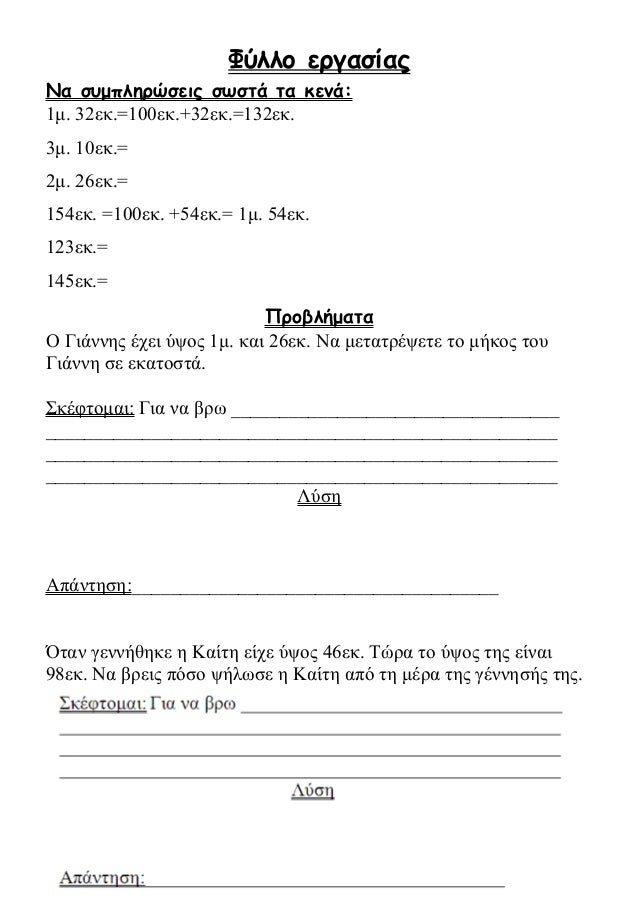Successfully reported this slideshow.
We use your LinkedIn profile and activity data to personalize ads and to show you more relevant ads. You can change your ad preferences anytime.Upcoming SlideShare
×

Μέτρηση μηκών με εκατοστά και χιλιοστά

1,564 views

Published on

Μέτρηση μηκών με εκατοστά και χιλιοστά

Published in: Education
• Full Name
Comment goes here.

Are you sure you want to Yes No
Your message goes here• Be the first to comment

• Be the first to like this

Μέτρηση μηκών με εκατοστά και χιλιοστά

1. 1. Φύλλο εργασίας Να συμπληρώσεις σωστά τα κενά: 1μ. 32εκ.=100εκ.+32εκ.=132εκ. 3μ. 10εκ.= 2μ. 26εκ.= 154εκ. =100εκ. +54εκ.= 1μ. 54εκ. 123εκ.= 145εκ.= Προβλήματα Ο Γιάννης έχει ύψος 1μ. και 26εκ. Να μετατρέψετε το μήκος του Γιάννη σε εκατοστά. Σκέφτομαι: Για να βρω __________________________________ _____________________________________________________ _____________________________________________________ _____________________________________________________ Λύση Απάντηση:______________________________________ Όταν γεννήθηκε η Καίτη είχε ύψος 46εκ. Τώρα το ύψος της είναι 98εκ. Να βρεις πόσο ψήλωσε η Καίτη από τη μέρα της γέννησής της.
2. 2. Ο Νικόλας βρήκε στον κήπο του σπιτιού του μια σειρά από 9 κάμπιες. Αν κάθε κάμπια έχει μήκος 3 εκατοστά, ποιο είναι το μήκος όλης της σειράς; Στοιχεία του προβλήματος Τι γνωρίζω Τι ψάχνω να βρω Λύση Απάντηση:_______________________________________ και λίγη επανάληψη… 4Χ8= 6Χ5= 2Χ4= 7Χ9= 9Χ2= 5Χ5= 6Χ7= 7Χ5= 3Χ9= 6Χ3= 8Χ2= 4Χ9= 8Χ3= 7Χ6= 5Χ8= 7Χ3= 7Χ5= 9Χ3= 7Χ4= 9Χ9= 9Χ8= 9Χ5- 8Χ9= 3Χ9= 8Χ5= 2Χ7= 5Χ7= 4Χ8= 9Χ4= 4Χ5= 6Χ9= 8Χ8= 9Χ6= 7Χ8= 8Χ4= 7Χ3= 10Χ4= 8Χ6= 6Χ8= 8Χ7= 9Χ7= 3Χ4= 6Χ6= 7Χ6= 5Χ6= 4Χ7= 7Χ7= 4Χ6= 5Χ9=